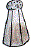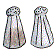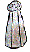Name:    2nd Grade Operations and Algebraic Thinking- Work with equal groups of objects to gain foundations for multiplication, Standard 4, Post Test 1 Use addition to find the total number of objects arranged in rectangular arrays with up to 5 rows and up to 5 columns; write an equation to express the total as a sum of equal addends.

Multiple Choice
Identify the choice that best completes the statement or answers the question.

1.

Which equation describes the array?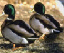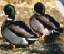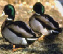a. 6 + 6 + 6 c. 4 + 4 + 4 b. 1 + 1 + 1 + 1 + 1 + 1 d. 3 + 3 + 3

2.

Which equation describes the array?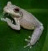a. 8 c. 1 + 4 + 1 + 4 b. 1 + 1 + 1 + 1 d. 2 + 2 + 2 + 2

3.

Which equation describes the array?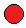a. 4 + 4 + 4 + 4 c. 1 + 4 + 1 + 4 b. 2 + 2 + 2 + 2 d. 1 + 1 + 1 + 1

4.

Which equation describes the array?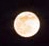a. 5 + 5 + 5 + 5 c. 1 + 4 + 1 + 4 b. 4 + 4 + 4 + 4 d. 4 + 4 + 4 + 4

5.

Which equation describes the array?a. 3 + 3 + 3 + 3 c. 5 + 5 + 5 + 5 b. 4 + 4 + 4 + 4 d. 4 + 5 + 4 + 5

Completion
Complete each statement.

6.

Write an equation for the array below. Leave a space between numbers and symbols.  DO NOT solve the problem.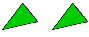7.

Write an equation for the array below. Leave a space between numbers and symbols.  DO NOT solve the problem.